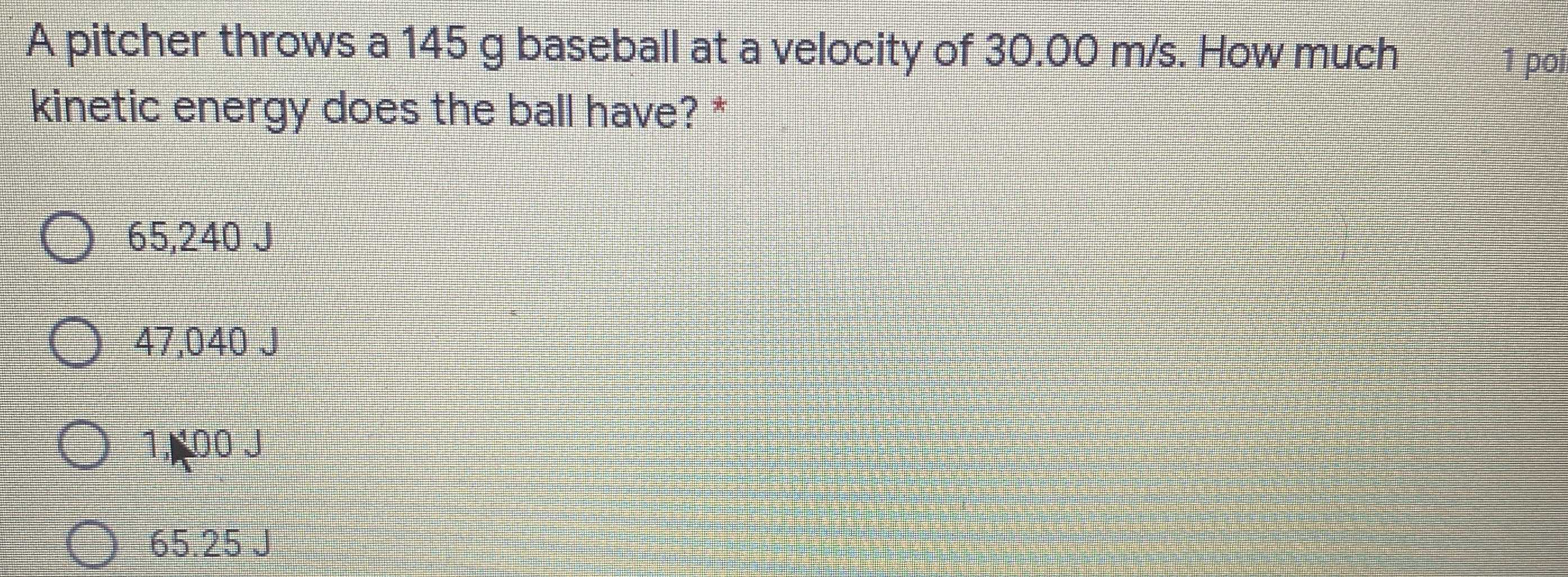### ¿Todavía tienes preguntas de matemáticas?

Pregunte a nuestros tutores expertos
Algebra
PreguntaA pitcher throws a $$145 g$$ baseball at a velocity of $$30.00 m / s$$ . How much kinetic energy does the ball have? $$^ { * }$$

$$65,240 J$$

$$47,040 J$$

$$1,400 J$$

$$65.25 J$$